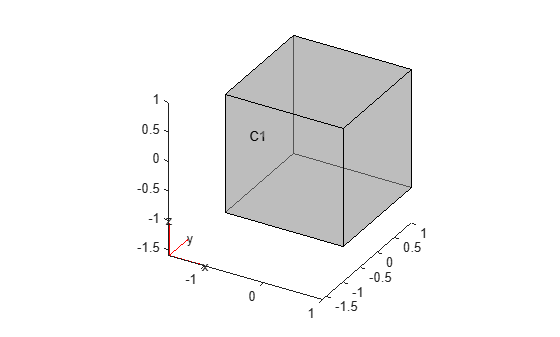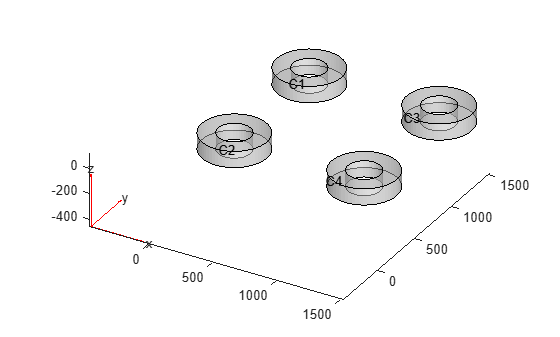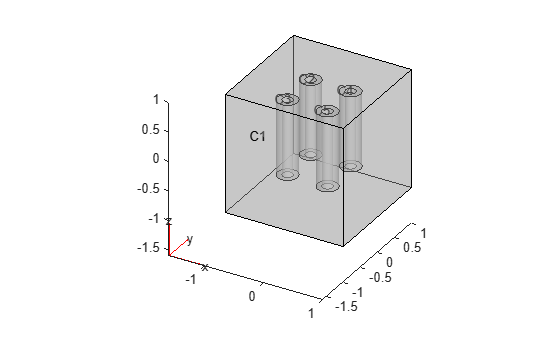Combine two geometries by adding one inside a cell of another

Since R2021a

## Syntax

``g3 = addCell(g1,g2)``

## Description

example

````g3 = addCell(g1,g2)` creates nonempty cells inside `g1` using all cells of `g2`. All cells of the geometry `g2` must be contained inside one cell of the geometry `g1`. Ensure that the geometries do not have enclosed cavities and do not intersect one another.The combined geometry contains cells from both geometries. The cells from `g1` retain their original IDs, while the cells from `g2` are numbered starting with `N+1`, where `N` is the number of cells in `g1`. NoteAfter modifying a geometry, always call `generateMesh` to ensure a proper mesh association with the new geometry. ```

## Examples

collapse all

Create and plot a geometry.

```g1 = multicuboid(2,2,2,"Zoffset",-1); pdegplot(g1,"CellLabels","on","FaceAlpha",0.5)```Import and plot another geometry.

```g2 = importGeometry("DampingMounts.stl"); pdegplot(g2,"CellLabels","on","FaceAlpha",0.5)```Scale and move the second geometry to fit entirely within the cube `g1`.

```g2 = scale(g2,[1/1500 1/1500 1/100]); g2 = translate(g2,[-0.5 -0.5 -0.5]);```

Plot the result.

`pdegplot(g2,"CellLabels","on","FaceAlpha",0.5)`Combine the geometries and plot the result. The combined geometry `g3` contains cells from both geometries. The cell from `g1` keeps its ID, and the cells from `g2` are now C2, C3, C4, and C5.

```g3 = addCell(g1,g2); pdegplot(g3,"CellLabels","on","FaceAlpha",0.4)```## Input Arguments

collapse all

3-D geometry, specified as an `fegeometry` object or a `DiscreteGeometry` object. For more information, see `fegeometry` and DiscreteGeometry Properties.

3-D geometry, specified as an `fegeometry` object or a `DiscreteGeometry` object.

## Output Arguments

collapse all

Resulting 3-D geometry, returned as an `fegeometry` object or a `DiscreteGeometry` object. If both `g1` and `g2` are `DiscreteGeometry` objects, the resulting geometry `g3` is also a `DiscreteGeometry` object. Otherwise, it is an `fegeometry` object.

## Version History

Introduced in R2021a

expand all